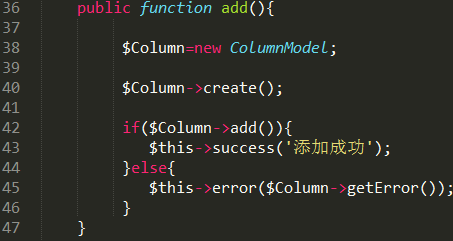# 使用ThinkPHP的自动完成实现无限级分类实例详解

更新时间：2016年09月02日 14:41:25   作者：TigerYangWTH1、模板2、控制器

・index模块3、模型以便于各位看官复制测试

1、模板

```<form action="__URL__/add" method="post">

<option value="0">栏目</option>
<volist name='list' id='vo'>
<option value="{\$vo['id']}">
{\$vo['blank']}{\$vo['name']}</option>
</volist>
</select><br/>

<input type="submit" value="添加"/>
</form>```

2、控制器

```<?php
class IndexAction extends Action
{
/**
+----------------------------------------------------------
* 默认操作
+----------------------------------------------------------
*/
public function index(){
\$Column=new ColumnModel();
\$list=\$Column->field("id,name,fid,sort,concat(sort,'-',id) nsort")->order('nsort asc')->select();
foreach(\$list as \$key=>\$val){
\$layer=count(explode('-',\$list[\$key]['nsort']));
\$list[\$key]['blank']='';
for(\$i=0;\$i<\$layer;\$i++){
if(\$i==\$layer-1){
\$list[\$key]['blank'].='---|';
}else{
\$list[\$key]['blank'].='---';
}
}
}
\$this->assign('list',\$list);
\$this->display();
}
\$Column=new ColumnModel;
\$Column->create();
\$this->success('添加成功');
}else{
\$this->error(\$Column->getError());
}
}
}
?>```

3、模型

```<?php
class ColumnModel extends Model{
protected \$_auto=array(
array('name','trim',0,'function'),//过滤用户不小心输入的空白字符
array('sort','createsort',0,'callback'),
);
/*
*自动完成sort字段
*根据POST过来的fid来查询上级sort，以确定本级sort
*/
protected function createsort(){
\$fid=\$_POST['fid']?(int)\$_POST['fid']:0;//如果用户没有选择父栏目，则默认父栏目id为0
if(\$fid!='0'){
\$list=\$this->where("id=\$fid")->find();
\$data=\$list['sort'].'-'.\$fid;
}else{
\$data='0';
}
return \$data;
}
}
?>```